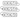# Remove nth Node from Last - Leet Code Solution

September 11, 2019## Problem Statement

Given a linked list, remove the n-th node from the end of list and return its head.

## Solution

There can be many solution for this. Lets start with a brute force simple solution.

### Algorithm-1

You can simply iterate full link list, and count its length. Lets say it: L Calculate L-n. And, move pointer from head to this many times. This node will be the one who has to be deleted.

This requires 2 iteration. Although complexity is still O(n). But, we can think of more optimized version of this algorithm.

### Algorithm-2

We can leverage two pointer concept. Where one pointer moves ahead of first pointer. We just need to keep a distance of ‘n’ between two pointers.

• Keep p1 at head, move p2 ahead one step at a time.
• Move p2 n times.
• Now, difference between p1 and p2 is ‘n’
• We now have to move both the pointers ahead with same speed. i.e. one step at a time.
• When 2nd pointer reaches end of link list, the first pointer will be at the desired node that we want to delete.

Lets look at the code:

``````public class ListNode {
int val;
ListNode next;
ListNode(int x) { val = x; }
}
public ListNode removeNthFromEnd(ListNode head, int n) {
ListNode prev = null;

for (int i=0; i<n; i++) {
fast = fast.next;
}
while (fast != null) {
prev = h;
h = h.next;
fast = fast.next;
}
if (prev == null) {
//special condition where first pointer is at the head only. And, we want to delete head
}

prev.next = h.next;
}``````

Above code does assume that length of list is always greater than equal to ‘n’.

## Performance

BTW, this code submission to leetcode turns out to be the fastest.

``````Runtime: 0 ms, faster than 100.00% of Java online submissions for Remove Nth Node From End of List.
Memory Usage: 34.8 MB, less than 100.00% of Java online submissions for Remove Nth Node From End of List.``````